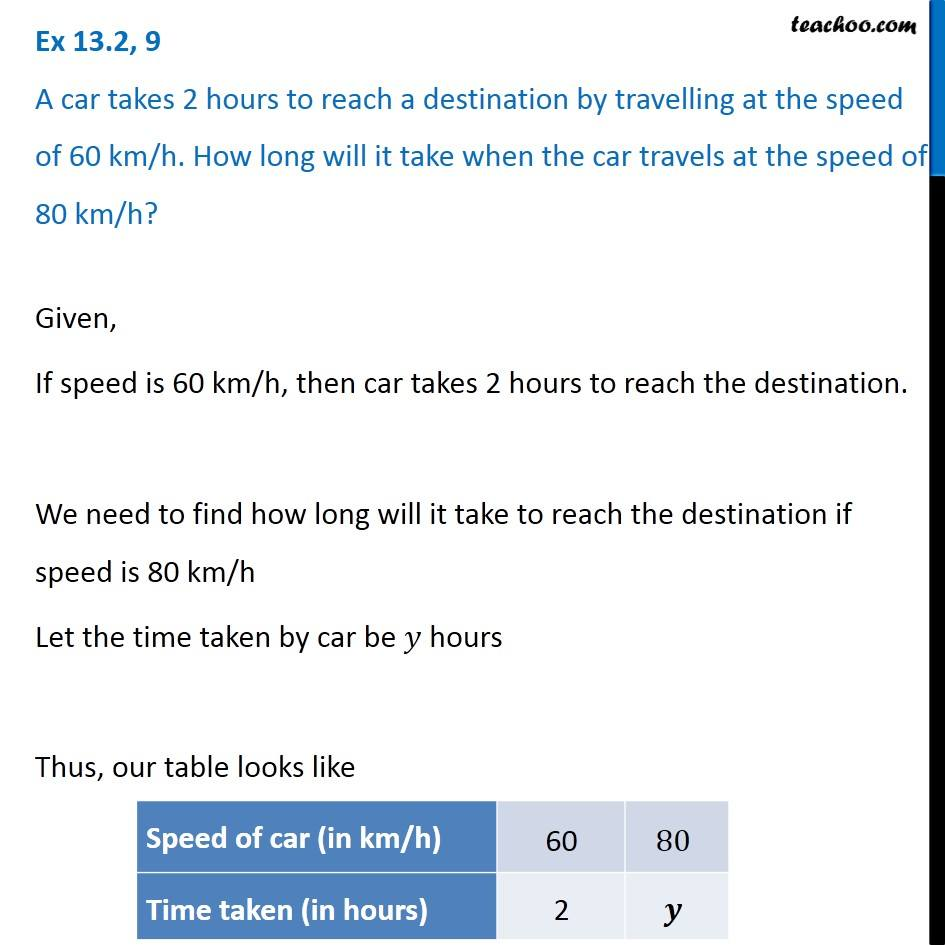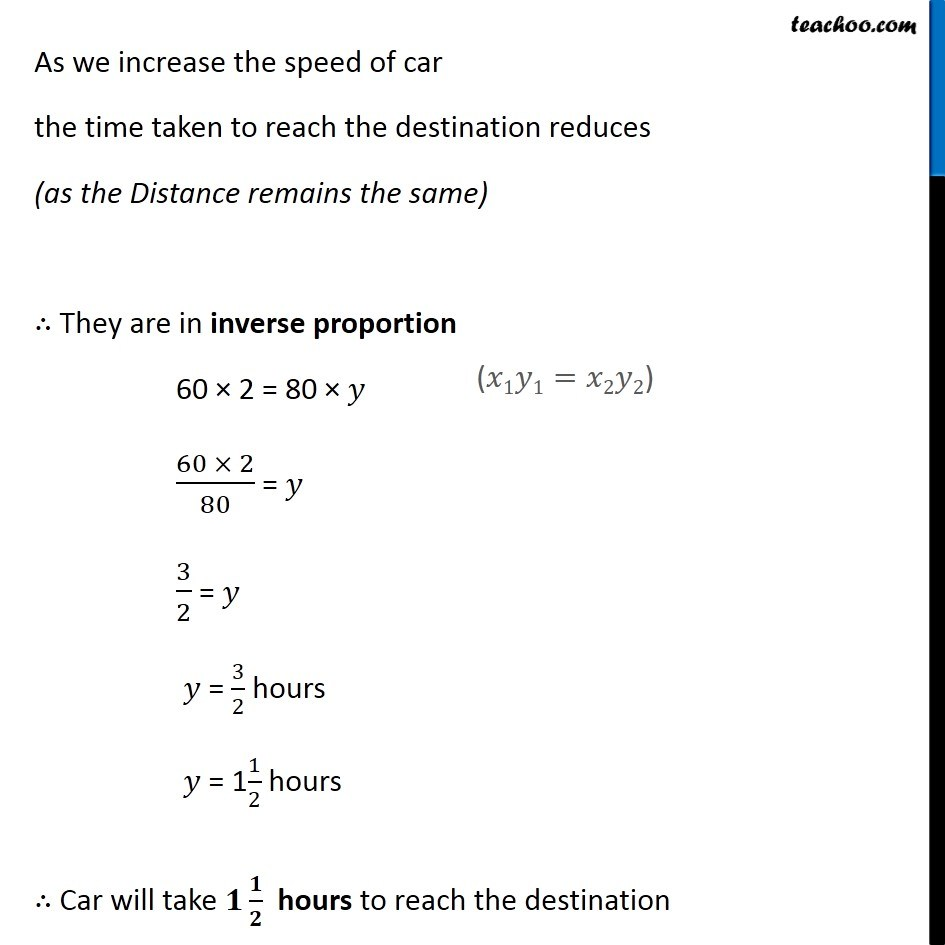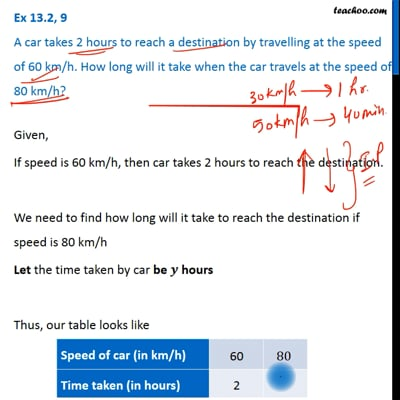Inverse Proportion

Chapter 11 Class 8 Direct and Inverse Proportions
Concept wiseThis video is only available for Teachoo black users

Learn in your speed, with individual attention - Teachoo Maths 1-on-1 Class

### Transcript

Ex 11.2, 9 A car takes 2 hours to reach a destination by travelling at the speed of 60 km/h. How long will it take when the car travels at the speed of 80 km/h? Given, If speed is 60 km/h, then car takes 2 hours to reach the destination. We need to find how long will it take to reach the destination if speed is 80 km/h Let the time taken by car be 𝑦 hours Thus, our table looks like As we increase the speed of car the time taken to reach the destination reduces (as the Distance remains the same) ∴ They are in inverse proportion 60 × 2 = 80 × 𝑦 (60 × 2)/80 = 𝑦 3/2 = 𝑦 𝑦 = 3/2 hours 𝑦 = 11/2 hours ∴ Car will take 𝟏 𝟏/𝟐 hours to reach the destination